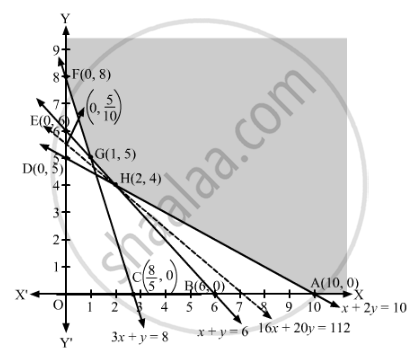# A Dietician Wishes to Mix Together Two Kinds of Food X and Y in Such a Way that the Mixture Contains at Least 10 Units of Vitamin A, 12 Units of Vitamin B and 8 Units of Vitamin C. - Mathematics

Sum

A dietician wishes to mix together two kinds of food X and Y in such a way that the mixture contains at least 10 units of vitamin A, 12 units of vitamin B and 8 units of vitamin C. The vitamin contents of one kg food is given below:

 Food Vitamin A Vitamin B Vitamin C X 1 2 3 Y 2 2 1

One kg of food X costs ₹16 and one kg of food Y costs ₹20. Find the least cost of the mixture which will produce the required diet?

#### Solution

Let x kg of food X and kg of food Y are mixed together to make the mixture.

The cost of food X is ₹16/kg and that of food Y is ₹20/kg. So, x kg of food X and y kg of food Y will cost ₹(16x + 20y).

Since one kg of food X contains 1 unit of vitamin A and one kg of food Y contains 2 units of vitamin A, therefore, x kg of food X and y kg of food Y will contain (x + 2y) units of vitamin A. But, the mixture should contain atleast 10 units of vitamin A.

∴ x + 2≥ 10

Similarly, x kg of food X and y kg of food Y will contain (2x + 2y) units of vitamin B. But, the mixture should contain atleast 12 units of vitamin B.

∴ 2x + 2y  ≥ 12

⇒ x + y  ≥ 6

Also, x kg of food X and y kg of food Y will contain (3x + y) units of vitamin C. But, the mixture should contain atleast 8 units of vitamin C.

∴ 3x + ≥ 8

Thus, the given linear programming problem is

Minimise Z = 16x + 20y

subject to the constraints

x + 2≥ 10

x + y  ≥ 6

3x + ≥ 8

x, y ≥ 0

The feasible region determined by the given constraints can be diagrammatically represented as,The coordinates of the corner points of the feasible region are A(10, 0), H(2, 4), G(1, 5) and F(0, 8).

The value of the objective function at these points are given in the following table.

 Corner Point Z = 16x + 20y (10, 0) 16 × 10 + 20 × 0 = 160 (2, 4) 16 × 2 + 20 × 4 = 112   → Minimum (1, 5) 16 × 1 + 20 × 5 = 116 (0, 8) 16 × 0 + 20 × 8 = 160

The smallest value of Z is 112 which is obtained at (2, 4).

It can be seen that the open half-plane represented by 16x + 20y < 112 has no common points with the feasible region.

So, the minimum value of Z is 112. Hence, the least cost of the mixture which will produce the required diet is ₹112.

Concept: Graphical Method of Solving Linear Programming Problems
Is there an error in this question or solution?

#### APPEARS IN

RD Sharma Class 12 Maths
Chapter 30 Linear programming
Exercise 30.3 | Q 14 | Page 40